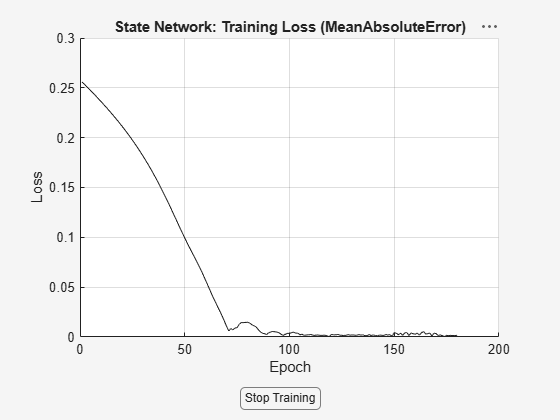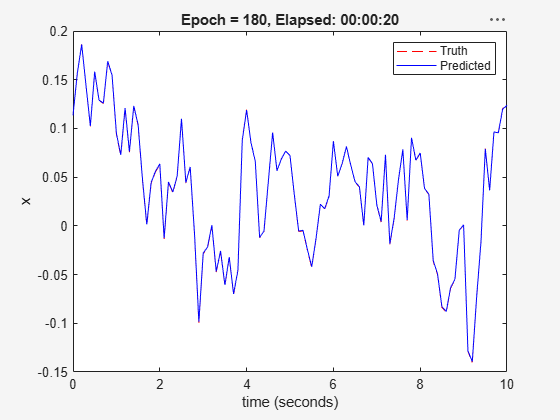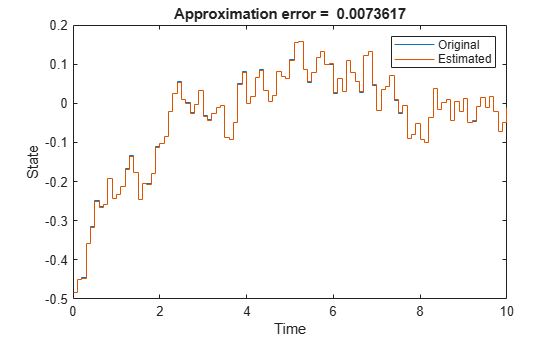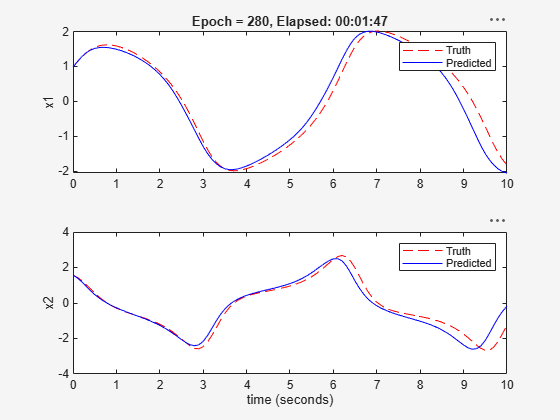# nlssest

Estimate nonlinear state-space model using measured time-domain system data

Since R2022b

## Syntax

``nssEstimated = nlssest(U,Y,nss)``
``nssEstimated = nlssest(Data,nss)``
``nssEstimated = nlssest(___,Options)``
``nssEstimated = nlssest(___,Name=Value)``

## Description

````nssEstimated = nlssest(U,Y,nss)` uses measured input and output data sets `U` and `Y`, and default training options, to train the state and output networks of the `idNeuralStateSpace` object `nss`. It returns the `idNeuralStateSpace` object `nssEstimated` with trained state and the output networks.```
````nssEstimated = nlssest(Data,nss)` uses measured input and output data stored in `Data`, and the default training options, to train the state and output networks of `nss`.```
````nssEstimated = nlssest(___,Options)` specifies custom training options, which use either the Adam algorithm or SGDM algorithm to train the networks.```

example

````nssEstimated = nlssest(___,Name=Value)` specifies name-value pair arguments after any of the input argument in the previous syntax. Use name-value pair arguments to specify whether you want to use the last experiment for validation, and the frequency for the validation plots.```

## Examples

collapse all

This example shows how to estimate a neural-state space model with one input and one state equal to the output. First, you collect identification and validation data by simulating a linear system, then use the collected data to estimate and validate a neural state-space system, and finally compare the estimated system to the original linear system used to produce the data.

Define Linear Model for Data Collection

Define a linear time-invariant discrete-time model that can be easily simulated to collect data. For this example, use a low-pass filter with a bandwidth of about `1` rad/sec, discretized with a sample time of `0.1` sec.

```Ts = 0.1; sys = ss(c2d(tf(1,[1 1]),Ts));```

The identification of a neural state-space system requires you to have measurement of the system states. Therefore, transform the state-space coordinates so that the output is equal to the state.

```sys.b = sys.b*sys.c; sys.c = 1;```

Generate Data Set for Identification

Fix the random generator seed for reproducibility.

`rng(0);`

Run `1000` simulations each starting at a different initial state and lasting `1` second. Each experiment must use identical time points.

```N = 1000; U = cell(N,1); Y = cell(N,1); for i=1:N % Each experiment uses random initial state and input sequence t = (0:Ts:1)'; u = 0.5*randn(size(t)); x0 = 0.5*randn(1,1); % Obtain state measurements over t x = lsim(sys,u,t,x0); % Each experiment in the data set is a timetable U{i} = array2timetable(u,RowTimes=seconds(t)); Y{i} = array2timetable(x,RowTimes=seconds(t)); end```

Generate Data Set for Validation

Run one simulation to collect data that will be used to visually inspect training result during the identification progress. The validation data set can have different time points. For this example, simulate the trained model for 10 seconds.

```% Use random initial state and input sequence t = (0:Ts:10)'; u = 0.5*randn(size(t)); x0 = 0.5*randn(1,1); % Obtain state measurements over t x = lsim(sys,u,t,x0); % Append the validation experiment (also a timetable) as the last entry in the data set U{end+1} = array2timetable(u,RowTimes=seconds(t)); Y{end+1} = array2timetable(x,RowTimes=seconds(t));```

Create a Neural State-Space Object

Create time-invariant discrete-time neural state-space object with one state identical to the output, one input, and sample time `Ts`.

`nss = idNeuralStateSpace(1,NumInputs=1,Ts=Ts);`

Configure State Network

Define the neural network that approximates the state function as having no hidden layer (because the output layer, which is a fully connected layer, should be sufficient to approximate the linear function: $x\left[k+1\right]=\mathit{Ax}\left[k\right]+\mathit{Bu}\left[k\right]$).

Use `createMLPNetwork` to create the network and dot notation to assign it to the `StateNetwork` property of `nss`.

```nss.StateNetwork = createMLPNetwork(nss,'state', ... LayerSizes=zeros(0,1), ... WeightsInitializer="glorot", ... BiasInitializer="zeros");```

Display the number of network parameters.

`summary(nss.StateNetwork)`
``` Initialized: true Number of learnables: 3 Inputs: 1 'x[k]' 1 features 2 'u[k]' 1 features ```

Specify the training options for the state network. Use the Adam algorithm, specify the maximum number of epochs as 3`00` (an epoch is the full pass of the training algorithm over the entire training set), and let the algorithm use the entire set of 1000 experiment data as a batch set to calculate the gradient at each iteration.

```opt = nssTrainingOptions('adam'); opt.MaxEpochs = 300; opt.MiniBatchSize = N;```

Also specify the `InputInterSample` option to hold the input constant between two sampling interval. Finally, specify the learning rate.

```opt.InputInterSample = "zoh"; opt.LearnRate = 0.01;```

Estimate the Neural State-Space System

Use `nlssest` to train the state network of `nss`, using the identification data set and the predefined set of optimization options.

`nss = nlssest(U,Y,nss,opt,'UseLastExperimentForValidation',true);````Generating estimation report...done. ```

The validation plot shows that the resulting system is able to reproduce well the validation data.

Compare the Estimated System to the Original Linear System

Define a random input and initial condition.

```x0 = 0.3*randn(1,1); u0 = 0.3*randn(1,1);```

Calculate the next state according to the linear system equation.

`sys.a*x0 + sys.b*u0`
```ans = 0.4173 ```

Evaluate `nss` at the point defined by `x0` and `u0`.

`evaluate(nss,x0,u0)`
```ans = 0.4176 ```

The value of the next state calculated by evaluating `nss` is close to the one calculated by evaluating the linear system equation.

Display the linear system.

`sys`
```sys = A = x1 x1 0.9048 B = u1 x1 0.09516 C = x1 y1 1 D = u1 y1 0 Sample time: 0.1 seconds Discrete-time state-space model. ```

Linearize `nss` around zero

`linearize(nss,0,0)`
```ans = A = x1 x1 0.9053 B = u1 x1 0.09488 C = x1 y1 1 D = u1 y1 0 Sample time: 0.1 seconds Discrete-time state-space model. ```

The linearized system matrices are close to the ones of the original system.

Perform an Extra Validation Check

Create input time series and a random initial state.

```t = (0:Ts:10)'; u = 0.5*randn(size(t)); x0 = 0.5*randn(1,1);```

Simulate both the linear and neural state-space system with the same input data, from the same initial state.

```% Simulate original system from x0 ylin = lsim(sys,u,t,x0); % Simulate neural state-space system from x0 simOpt = simOptions('InitialCondition',x0); yn = sim(nss,array2timetable(u,RowTimes=seconds(t)),simOpt);```

Note that you can also use the following code to simulate `nss`.

```x = zeros(size(t)); x(1)=x0; for k = 1:length(t)-1, x(k+1) = evaluate(nss,x(k),u(k)); end ```

Plot the outputs of both systems, also display the norm of the difference in the plot title.

```stairs(t,[ylin yn.Variables]); xlabel("Time"); ylabel("State"); legend("Original","Estimated"); title(['Approximation error = ' num2str(norm(ylin-yn.Variables)) ])```The two outputs are similar, confirming that the identified system is a good approximation of the original one.

This example shows how to estimate a nonlinear neural-state space model with no inputs and a two-dimensional continuous state equal to the output. First, you collect identification and validation data by simulating a Van Der Pol system, then use the collected data to estimate and validate a neural state-space system, and finally compare the estimated system to the original system used to produce the data.

Define Model for Data Collection

Define a time-invariant continuous-time autonomous model that can be easily simulated to collect data. For this example, use an unforced Van der Pol oscillator (VDP) system, which is an oscillator with nonlinear damping that exhibits a limit cycle.

Specify the state equation using an anonymous function, using a damping coefficient of `1`.

`dx = @(x) [x(2); 1*(1-x(1)^2)*x(2)-x(1)];`

Generate Data Set for Identification

Fix the random generator seed for reproducibility.

`rng(0);`

Run 1000 simulations each starting at a different initial state and lasting 2 seconds. Each experiment must use identical time points.

```N = 1000; t = (0:0.1:2)'; Y = cell(1,N); for i=1:N % Create random initial state within [-2,2] x0 = 4*rand(2,1)-2; % Obtain state measurements over t (solve using ode45) [~, x] = ode45(@(t,x) dx(x),t,x0); % Each experiment in the data set is a timetable Y{i} = array2timetable(x,RowTimes=seconds(t)); end```

Generate Data Set for Validation

Run one simulation to collect data that will be used to visually inspect training result during the identification progress. The validation data set can have different time points. For this example, use the trained model to predict VDP behavior for 10 seconds.

```% Create random initial state within [-2,2] t = (0:0.1:10)'; x0 = 4*rand(2,1)-2; % Obtain state measurements over t (solve using ode45) [~, x] = ode45(@(t,x) dx(x),t,x0); % Append the validation experiment (also a timetable) as the last entry in the data set Y{end+1} = array2timetable(x,RowTimes=seconds(t));```

Create a Neural State-Space Object

Create time-invariant continuous-time neural state-space object with a two-element state vector identical to the output, and no input.

`nss = idNeuralStateSpace(2);`

Configure State Network

Define the neural network that approximates the state function as having two hidden layers with 8 neurons each, and hyperbolic tangent activation function.

Use `createMLPNetwork` to create the network and dot notation to assign it to the `StateNetwork` property of `nss`.

```nss.StateNetwork = createMLPNetwork(nss,'state', ... LayerSizes=[12 12], ... Activations="tanh", ... WeightsInitializer="glorot", ... BiasInitializer="zeros");```

Display the number of network parameters.

`summary(nss.StateNetwork)`
``` Initialized: true Number of learnables: 218 Inputs: 1 'x' 2 features ```

Specify the training options for the state network. Use the Adam algorithm, specify the maximum number of epochs as `400` (an epoch is the full pass of the training algorithm over the entire training set), and let the algorithm use the entire set of `1000` experiments as a batch set to calculate the gradient at each iteration.

```opt = nssTrainingOptions('adam'); opt.MaxEpochs = 400; opt.MiniBatchSize = N;```

Also specify the leaning rate.

`opt.LearnRate = 0.005;`

Estimate the Neural State-Space System

Use `nlssest` to train the state network of `nss`, using the identification data set and the predefined set of optimization options.

`nss = nlssest([],Y,nss,opt,'UseLastExperimentForValidation',true);````Generating estimation report...done. ```

The validation plot shows that the resulting system is able to reproduce well the validation data.

Compare the Estimated System to the Original Linear System

Define a random initial condition.

`x0 = 0.3*randn(2,1);`

Calculate the state derivative according to the original system equation.

`dx(x0)`
```ans = 2×1 0.3111 0.3243 ```

Evaluate `nss` at `x0`.

`evaluate(nss,x0)`
```ans = 2×1 0.3219 0.3271 ```

The value of the state derivative calculated by evaluating `nss` is close to the one calculated by evaluating the original system equation.

You can linearize `nss` around an operating point, and apply linear control analysis and synthesis methods on the resulting linear time-invariant state-space system.

`sys = linearize(nss,x0)`
```sys = A = x1 x2 x1 0.02485 1.13 x2 -0.9892 1.055 B = Empty matrix: 2-by-0 C = x1 x2 y1 1 0 y2 0 1 D = Empty matrix: 2-by-0 Continuous-time state-space model. ```

Simulate the neural state-space system

Create a time sequence and a random initial state.

```t = (0:0.1:10)'; x0 = 0.3*randn(2,1);```

Simulate both the original and neural state-space system with the same input data, from the same initial state.

```% Simulate original system from x0 [~, x] = ode45(@(t,x) dx(x),t,x0); % Simulate neural state-space system from x0 simOpt = simOptions('InitialCondition',x0,'OutputTimes',t); xn = sim(nss,zeros(length(t),0),simOpt);```

Plot the outputs of both systems, also display the norm of the difference in the plot title.

```figure; subplot(2,1,1) plot(t,x(:,1),t,xn(:,1)); ylabel("States(1)"); legend("Original","Estimated"); title(['Approximation error = ' num2str(norm(x-xn)) ]) subplot(2,1,2) plot(t,x(:,2),t,xn(:,2)); xlabel("Time"); ylabel("States(2)"); legend("Original","Estimated");```The two outputs are similar, confirming that the identified system is a good approximation of the original one.

## Input Arguments

collapse all

Input data. Specify `U` as:

• A timetable containing a variable for each input. The variable names of the timetable must match the input names of `nss`, and its row times must be `duration` objects. This timetable represents a single experiment. For more information, see `timetable` and `duration`.

• A double matrix with one column for each input signal and one row for each time step. Use this option only if the system is discrete-time (that is `nss.Ts` is greater than zero). This matrix represents a single experiment.

• A cell array of N experiments composed of timetables or double matrices. All the experiments must contain the same time points. In other words the time vector corresponding to all the observations must match.

• An empty array, `[]`, if `nss` has no inputs (that is `size(nss,2)` is zero).

Output data. Specify `Y` as:

• A timetable containing a variable for each output. The variable names of the timetable must match the output names of `nss`, and its row times must be `duration` objects. This timetable represents a single experiment. For more information, see `timetable` and `duration`.

• A double matrix with one column for each output signal and one row for each time step. Use this option only if the system is discrete-time (that is `nss.Ts` is greater than zero). This matrix represents a single experiment.

• A cell array of N experiments composed of timetables or double matrices. All the experiments must contain the same time points. In other words the time vector corresponding to all the observations must match.

Note

The first nx channels in `Y` must be state measurements (here, nx is the number of states specified in `nss`).

Input and output data, specified as a `timetable` or `iddata` object. This argument allows you to specify the training data using a single input argument rather than separate `U` and `Y` arguments. Specify `Data` as one of the following:

• An `iddata` object. If you have multiple experiments, create a multi-experiment `iddata` object. Use this option if all the input and output variables in an experiment share the time vector. For more information, see `merge (iddata)`.

• A timetable. The timetable must contain a variable for each of the input and output variables in `nss`. In the multi-experiment case, use a cell array of timetables. All the timetables in the cell array must use the same time vector.

Neural state-space system, specified as an `idNeuralStateSpace` object.

Training options, specified as an `nssTrainingADAM` or `nssTrainingSGDM` object. Create the training options set object options using the `nssTrainingOptions` command. If `nss` contains a non-state output network (that is, if `nss.OutputNetwork` contains two networks), you can pick different training options for the state transition function network, `nss.StateNetwork`, and the nontrivial output function `nss.OutputNetwork(2)`. Note that `nss.OutputNetwork(1)` does not contain any learnable parameters because is always fixed to the identity function returning all the states as outputs.

Example: `myNrlSS`

### Name-Value Arguments

Specify optional pairs of arguments as `Name1=Value1,...,NameN=ValueN`, where `Name` is the argument name and `Value` is the corresponding value. Name-value arguments must appear after other arguments, but the order of the pairs does not matter.

Example: `UseLastExperimentForValidation = true`

Option to use the last experiment for validation, specified as one of the following:

• `true` — The last experiment is not used for training, but only to display a validation plot after a number of epochs specified by `ValidationFrequency`. This allows you to monitor the estimation performance during the training process. The last experiment can have a different duration than all the other experiments

• `false` — All experiments are used for training, and no validation plot is displayed.

Example: `false`

Validation frequency, specified as a positive integer. This is the number of epochs after which the validation plot is updated with a new comparison (new predicted output against measured outputs).

Example: `ValidationFrequency=20`

## Output Arguments

collapse all

Estimated neural state-space system, returned as an `idNeuralStateSpace` object.

## Version History

Introduced in R2022b